Question

Four capacitors are arranged in the circuit shown in the figure. The capacitors have the values C1 = 27.5 μF, C2 = 45.5 μF, C3 = 50.5 μF, C4 = 40.5 μF, and the power supply is at voltage V = 16.5 V. What is the equivalent capacitance of the circuit?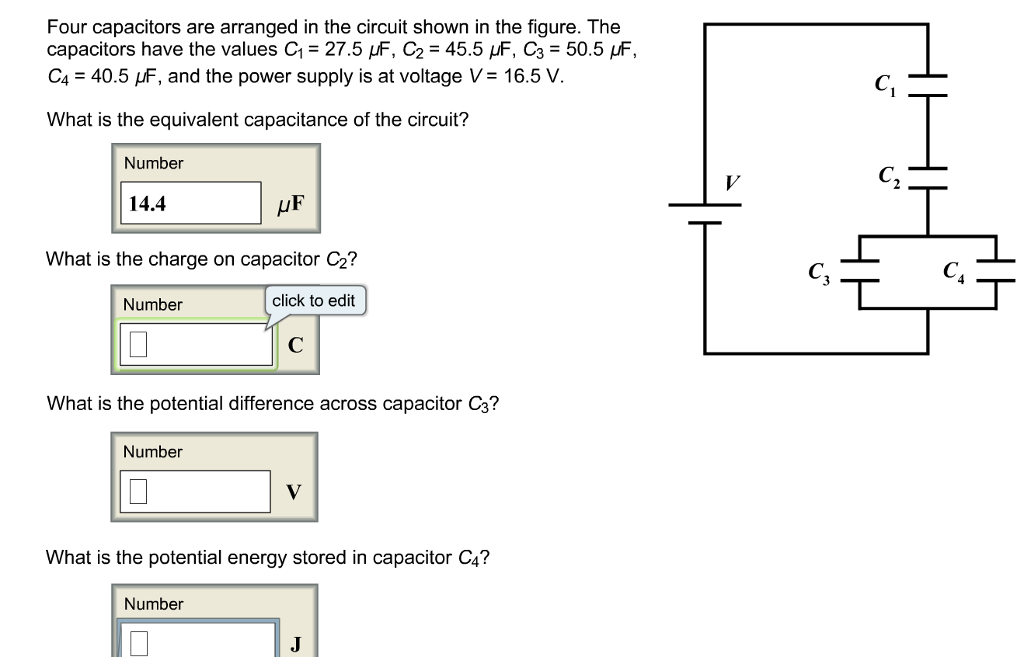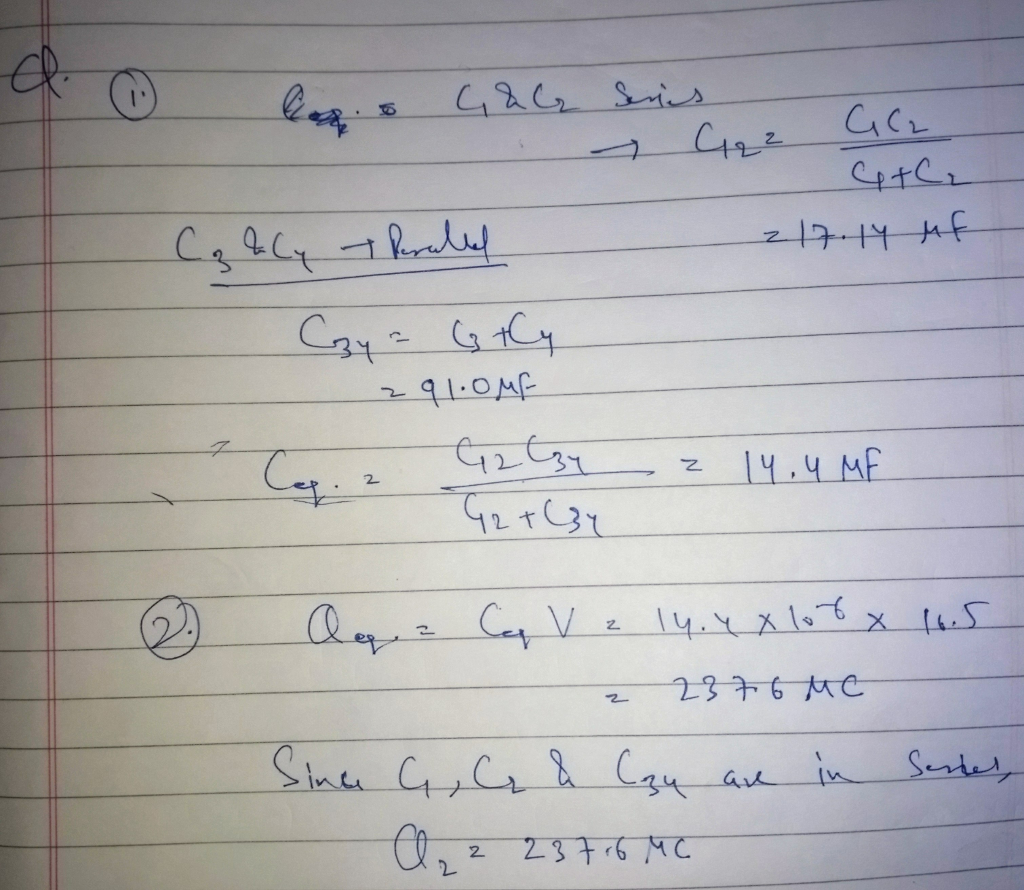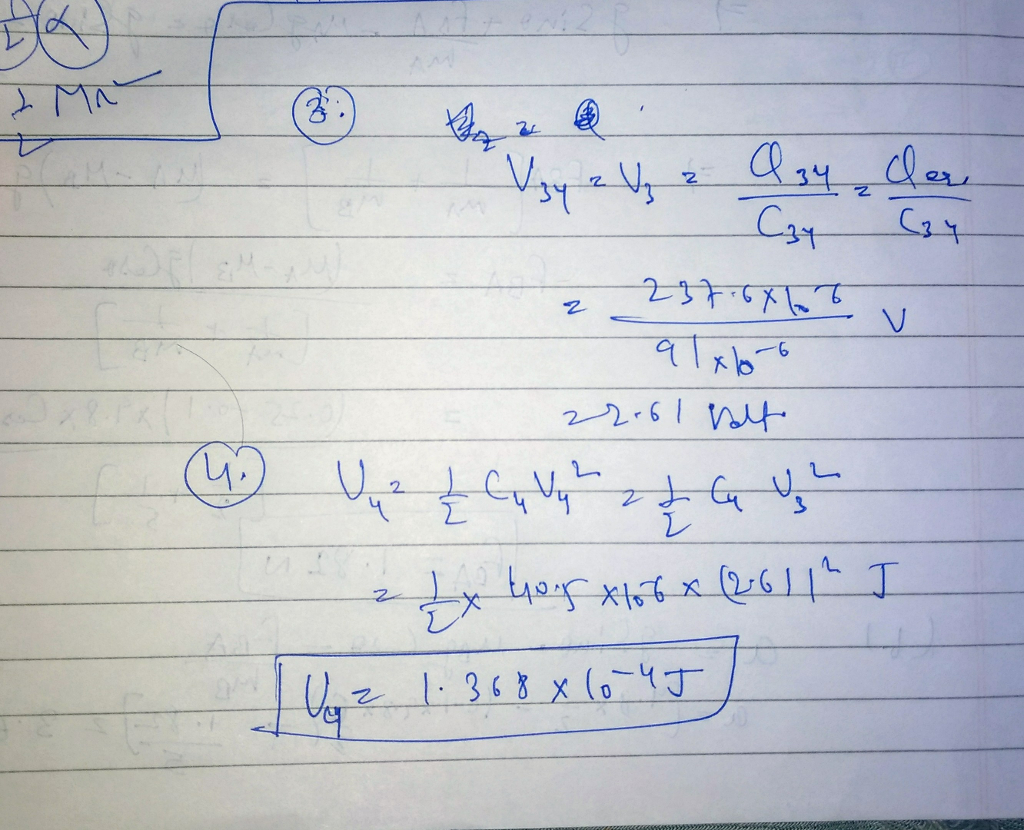#### Earn Coins

Coins can be redeemed for fabulous gifts.

Similar Homework Help Questions
• ### Four capacitors are arranged in the circuit shown in the figure. The capacitors have the values...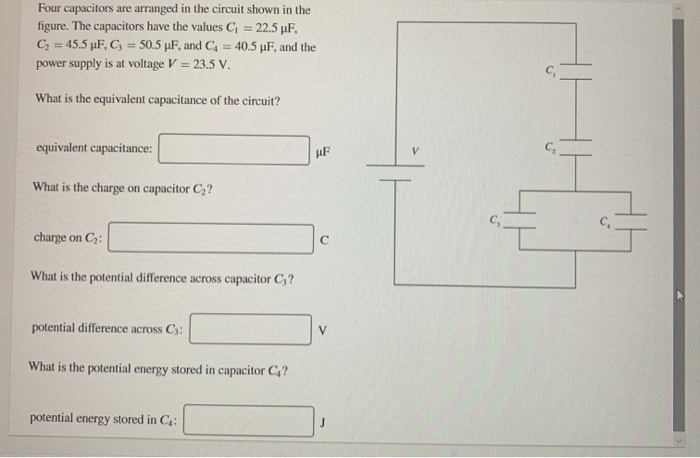Four capacitors are arranged in the circuit shown in the figure. The capacitors have the values G = 22.5 UF, C = 45.5 pF, C; = 50.5 pF, and Cd = 40.5 pF, and the power supply is at voltage V = 23.5 V. What is the equivalent capacitance of the circuit? equivalent capacitance: What is the charge on capacitor C2? charge on C: What is the potential difference across capacitor C;? potential difference across C3: What is the potential...

• ### 20 of 22 > Four capacitors are arranged in the circuit shown in the figure. The...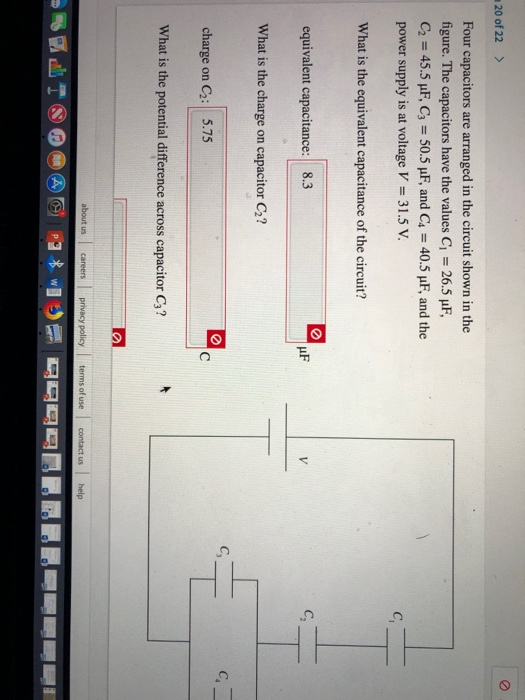20 of 22 > Four capacitors are arranged in the circuit shown in the figure. The capacitors have the values C 26.5 uF, C2 45.5 uF, C3 50.5 pF, and C4 40.5 uF, and the power supply is at voltage V 31.5 V C What is the equivalent capacitance of the circuit? C equivalent capacitance: 8.3 V What is the charge capacitor C2? on C. charge C2: 5.75 C on What is the potential difference across capacitor C3? about us...

• ### A circuit is constructed with five capacitors and a battery as shown. The values for the...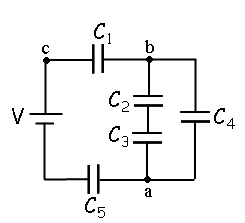A circuit is constructed with five capacitors and a battery as shown. The values for the capacitors are: C1 = C5 = 4.2 μF, C2 = 1 μF, C3 = 5.8 μF, and C4 = 4.4 μF. The battery voltage is V = 12 V. 1) What is Cab, the equivalent capacitance between points a and b?μF 2) What is Cac, the equivalent capacitance between points a and c? 3) What is Q5, the charge on capacitor C5?μC 4) What...

• ### four capacitors are arranged as in the figure, where C1= 3μF C2= 2μF, C3= 8μF and...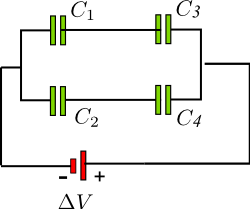four capacitors are arranged as in the figure, where C1= 3μF C2= 2μF, C3= 8μF and C4= 4μF. Potential Difference is ΔV = 90 V Find the equivalent capacitance of the system the charge on C1, the charge on C2 what is the potential Difference across C3, C4?

• ### A circuit is constructed with five capacitors and a battery as shown. The values for...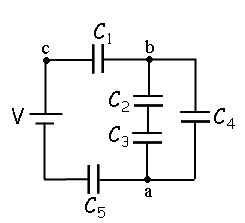A circuit is constructed with five capacitors and a battery as shown. The values for the capacitors are: C1 = C5 = 3.9 μF, C2 = 1.9 μF, C3 = 7.6 μF, and C4 = 2.2 μF. The battery voltage is V = 12 V. 4)What is Q2, the charge on C2? 5)What is Q1, the charge on capacitor C1? C1 b HH Cz C4 Ca HH a C5

• ### Consider the circuit as shown. The power supply has a voltage V = 34 V and...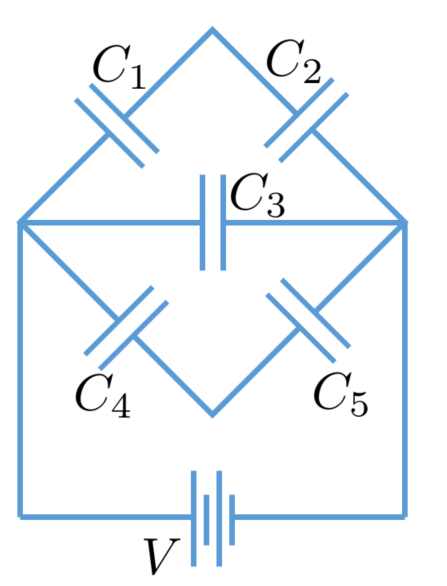Consider the circuit as shown. The power supply has a voltage V = 34 V and the capacitors have capacitance C1 = C2 = C3 = C4 = 80 µF and C5 = 208 µF. (a) What is the equivalent capacitance of this circuit? (b) What is the charge on capacitor C3? (c) What is the voltage on capacitor C3? (d) What is the charge on capacitor C5? (e) What is the voltage on capacitor C5?

• ### The circuit shown in the figure contains four capacitors whose values are as follows: C1=6 F,...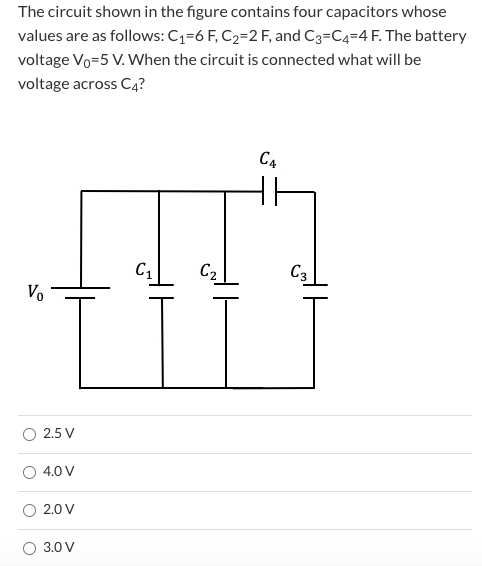The circuit shown in the figure contains four capacitors whose values are as follows: C1=6 F, C2=2 F, and C3=C4=4 F. The battery voltage Vo=5 V. When the circuit is connected what will be voltage across C4? C4 C1 C2 C3 V 2.5 V 4.0 V 2.0 V 3.0 V

• ### The circuit shown in the figure contains four capacitors whose values are as follows: C1=6 F,...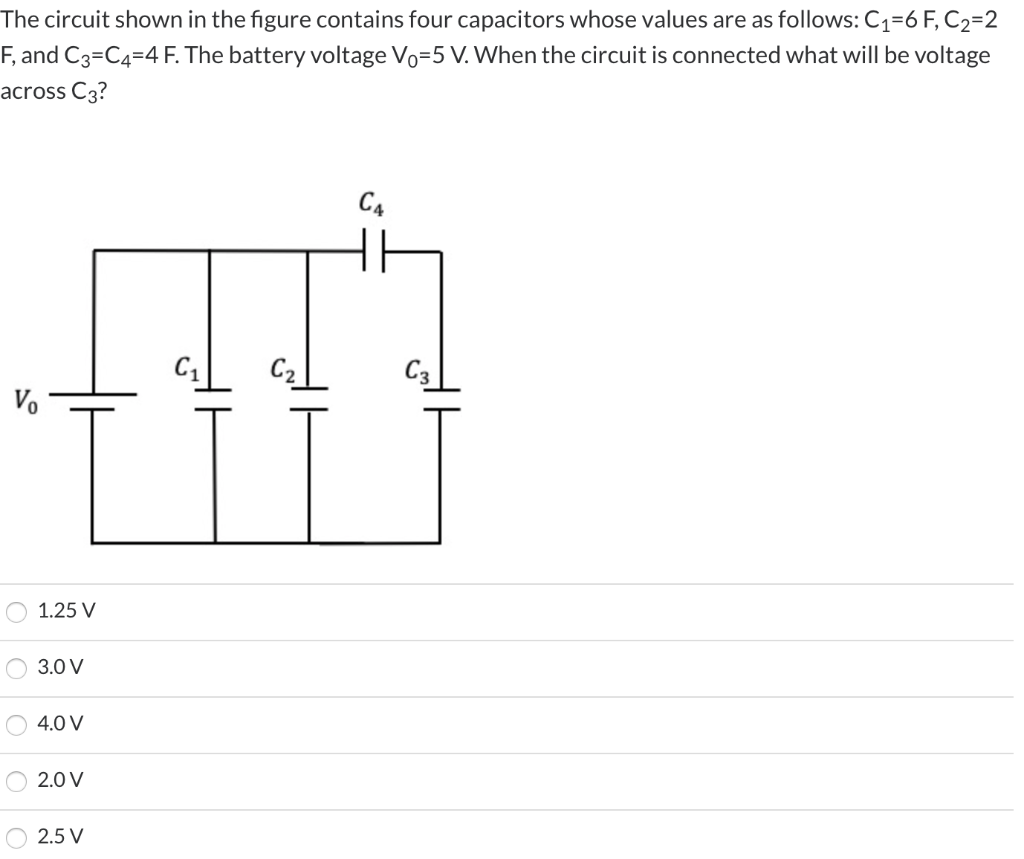The circuit shown in the figure contains four capacitors whose values are as follows: C1=6 F, C2=2 F, and C3=C4=4 F. The battery voltage Vo=5 V. When the circuit is connected what will be voltage across C3? C4 C1 C2 C3 1.25 V 3.0 V 4.0 V 2.0 V 2.5 V

• ### The circuit shown in the figure contains four capacitors whose values are as follows: C1=6 F,...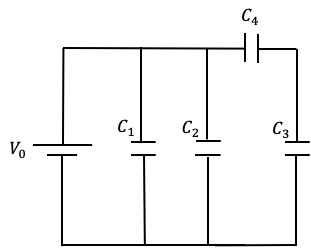The circuit shown in the figure contains four capacitors whose values are as follows: C1=6 F, C2=2 F, and C3=C4=4 F. The battery voltage V0=5 V. When the circuit is connected what will be charge on C3? C4 C1 C2 C3 G V.

• ### The circuit shown in the figure contains four capacitors whose values are as follows: C1=6 F,...The circuit shown in the figure contains four capacitors whose values are as follows: C1=6 F, C2=2 F, and C3=C4=4 F. The battery voltage V0=5 V. When the circuit is connected what will be voltage across C3? 1.25 V 3.0 V 4.0 V 2.0 V 2.5 V C4 C1 C2 C3 G V.